Home > ACC7 > Chapter cc38 > Lesson cc38.2.2 > Problem8-66

8-66.
1. Simplify each expression. Homework Help ✎

1.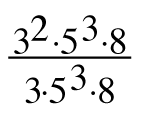2. (3x)4

3.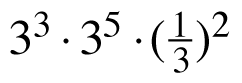4.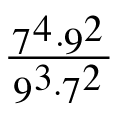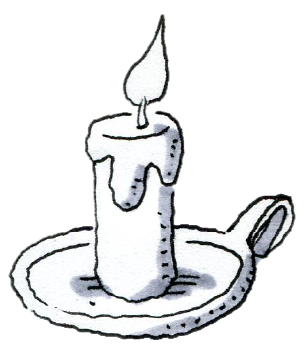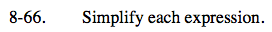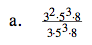Recall that when you divide a number by itself, the quotient is 1. Can you find any factors that are in both the numerator and the denominator?

You can rewrite the expression.

$\frac{3\cdot3\cdot5\cdot5\cdot5\cdot8}{3\cdot5\cdot5\cdot5\cdot8}$

There are three 5's on the top and on the bottom, which may be removed by division. Similarly, there are 8's and 3's that can be removed.

3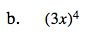Rewrite the expression and simplify.

(3x)(3x)(3x)(3x)

81x4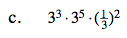You can rewrite this expression as:

$\frac{3^{3}3^{5}}{3^{2}}$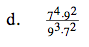See (a).